# Suspension Mechanics

## 1. Introduction

We examine the displacement of the wishbones by the effect of a sinusoidal excitation. An important part of the shock absorber is the wishbones, which is located at the wheel. Nowadays they are manufactured in many design, but the effect of the main loads is almost the same in all. Next, we will count on a general and simple arm.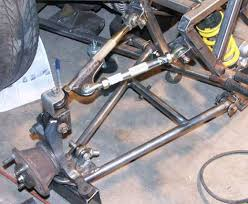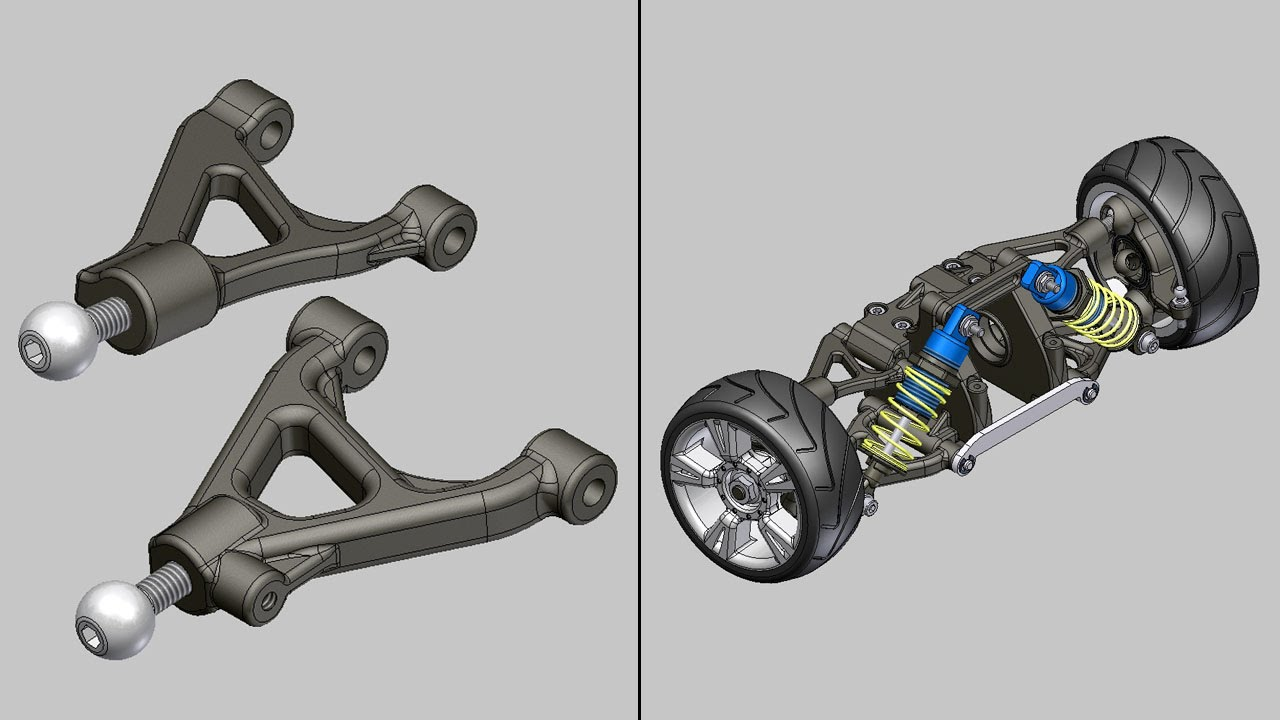## 2. Running the case

The command line to run this case is

``mpirun -np 4 feelpp_toolbox_solid --case "github:{repo:toolbox,path:examples/modules/csm/examples/suspension}"``
 The report of the execution of the command above is available here.

## 3. Data files

The case data files are available in Github here

## 4. Model/Geometry

The first step is to create the model of the object, which we can simply do in the Creo Parametric program. With this program was the fastest and easiest way to create the model.

The finished geometry (Creo) and the meshed model (Gmsh):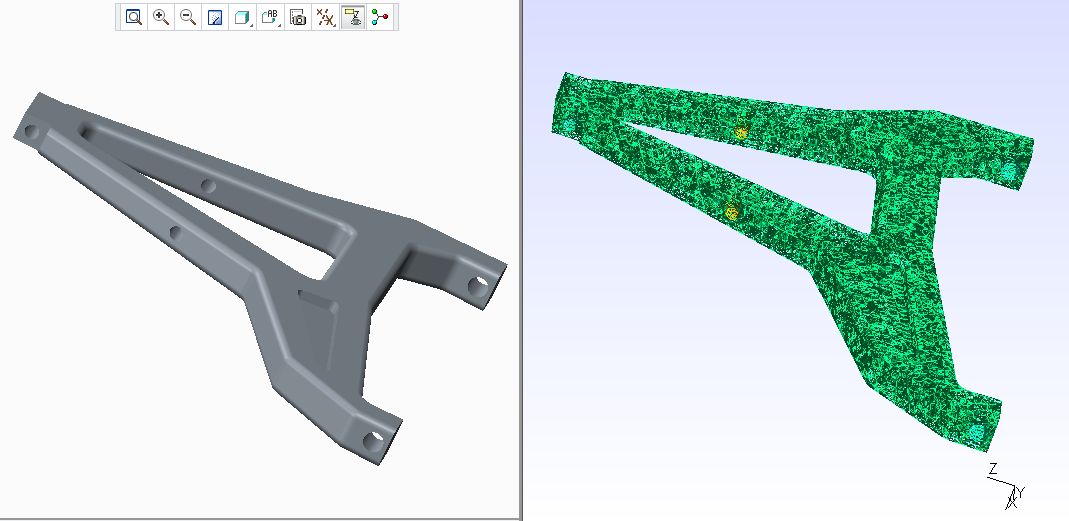## 5. Materials and boundary conditions

### 5.1. Materials

The beam is made of steel, so we need to use the following data:

• E = 200 GPa → 200e3 N/mm2

• nu = 0.3

• rho = 7870 kg/m3 → 7870e-9 kg/mm3

### 5.2. Boundary conditions

 The object is fixed at the ends of the holes. The holes in the middle have the force.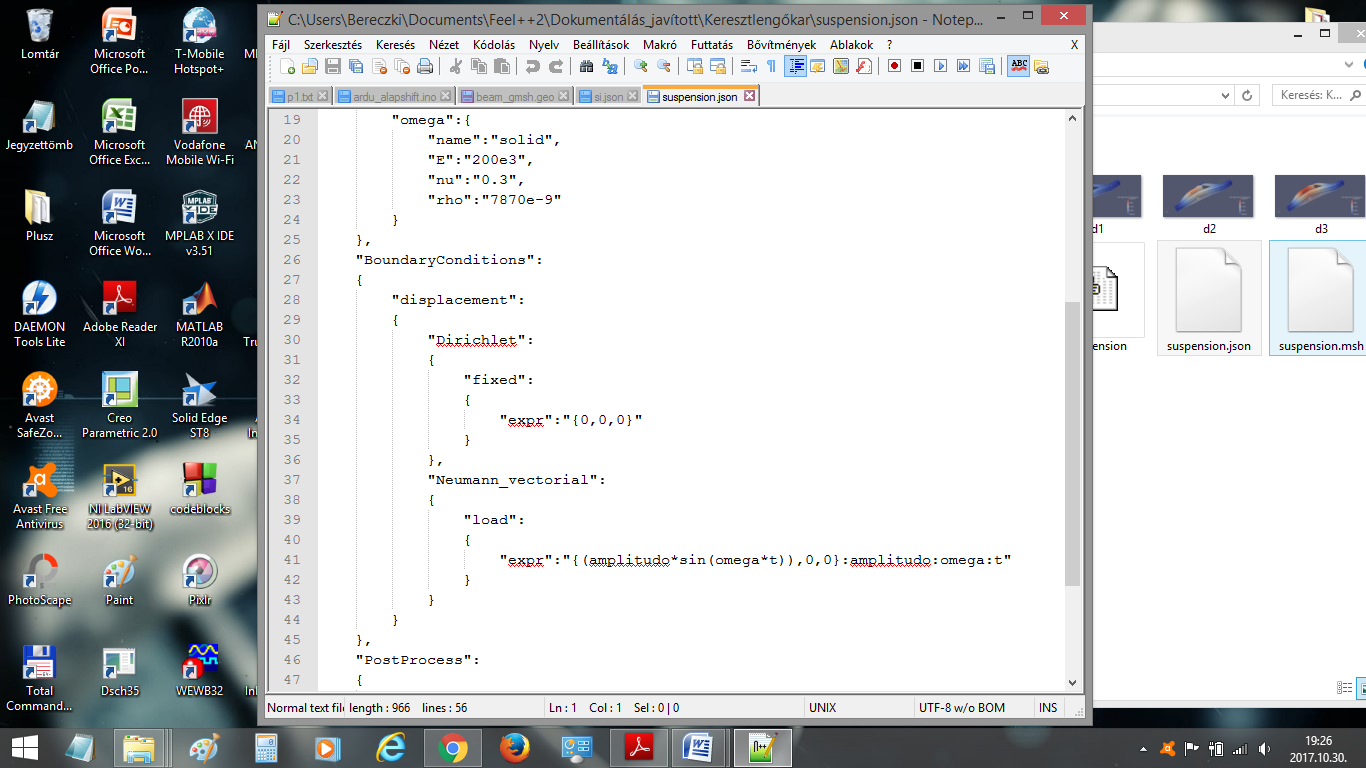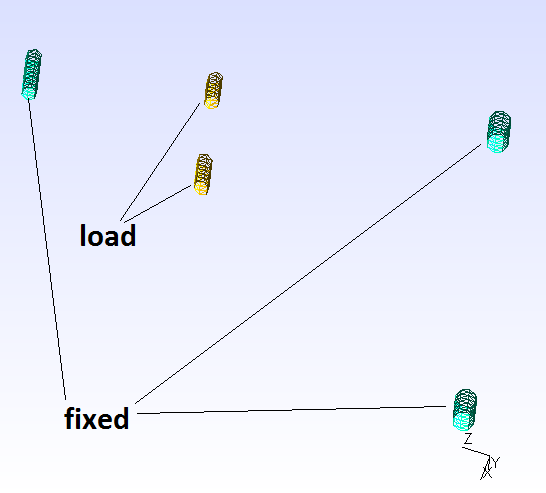## 6. Results

 The result were run in time (two whole period, but in the pictures can be seen only a quarter period).

### 6.1. Displacement

We display the displacement in the figures below. A 20x scale factor is used to make the displacement easy to see. The maximum displacement is 1.778 mm. The design of the wishbones is very good because there is only little displacement due to the force.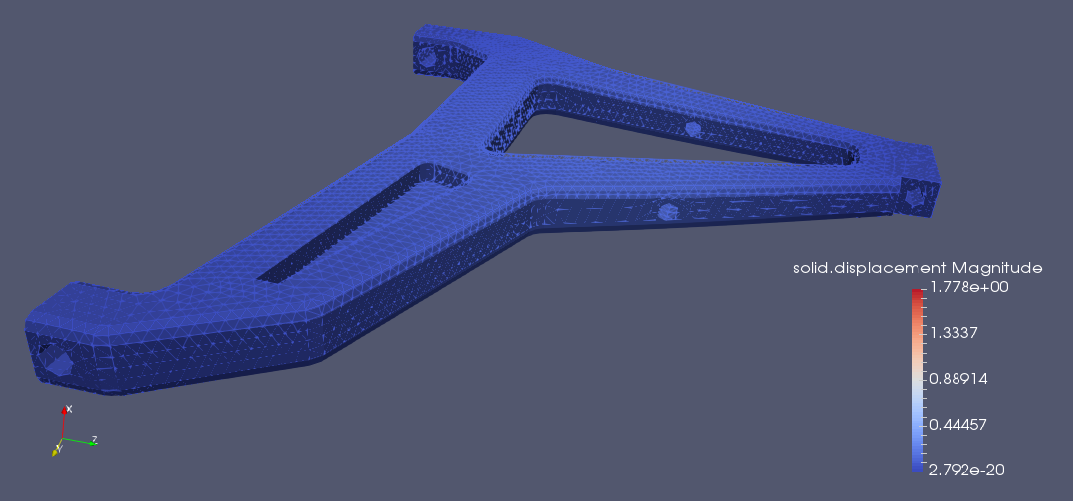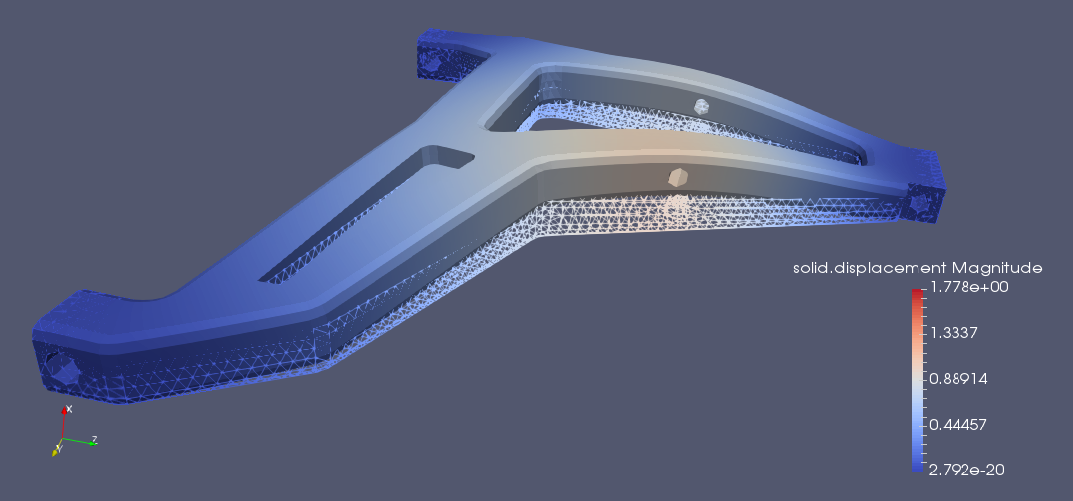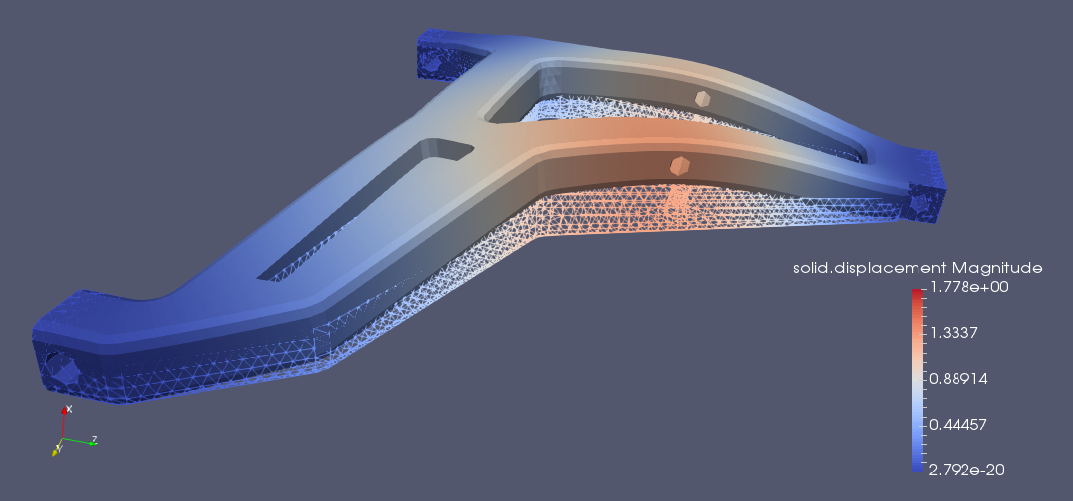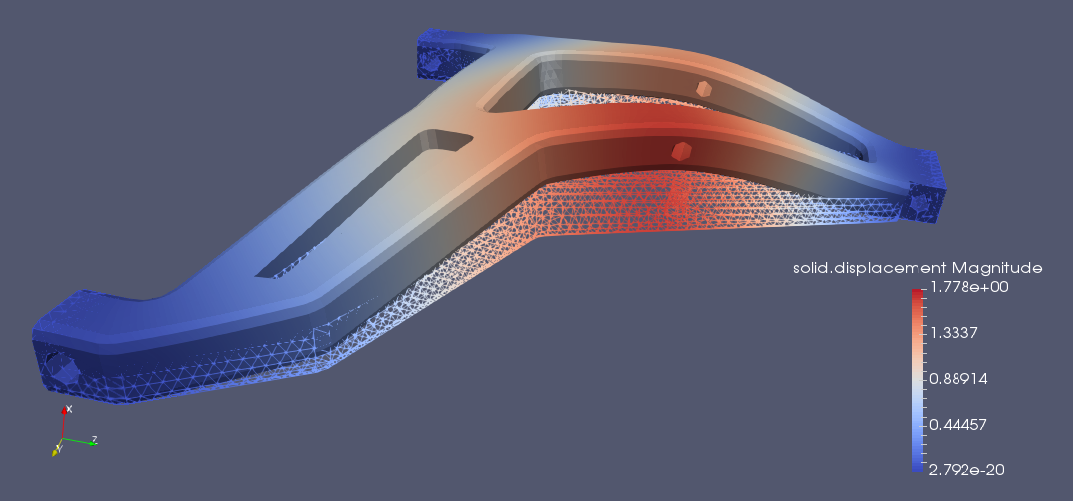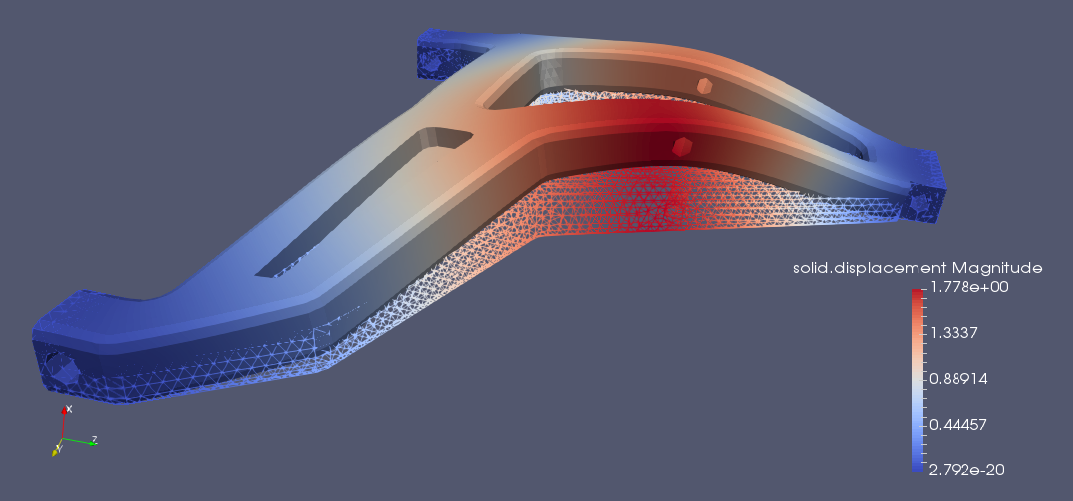3D interactive model

Finally, we provide a 3D interactive model of the fishbone